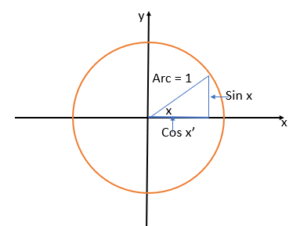# Sine Function

## What is a Sine Function?

The sine function (sin x) is a wave-like function, sometimes called a sine wave or sunusoid. It’s type of trigonometric function and is also classified as a periodic function. Trigonometric functions are functions involving angles while periodic functions repeat at set intervals.

It is sometimes called the real sine function to set it apart from its complex-valued counterpart.

For the cardinal sine function, see: Sinc Function.

## Trigonometric Meaning

The sine function is trigonometric, which means that it tells you something about circles and angles.

One way to define the sine function is in terms of a triangle. The sine tells you the vertical coordinate of an arc’s endpoint on a unit circle.

In a right angle, the sine is a ratio: the length of the opposite side (O), divided by the hypotenuse. You may remember the familiar acronym SOHCAHTOA: The first part (SOH) deals with the sine: Sine = Opposite / Hypotenuse.

The relationship between the sine and the other parts of a right angle can best explained with a graph:x is an angle (measured counterclockwise from the horizontal axis) along a unit circle‘s arc. Sin x gives you the vertical coordinate of the arc’s endpoint.

## Real Life Examples

The sine function has many real life applications, a few of which are:

• Triangulation, used in GPS-equipped cellphones,
• Musical notes,
• Submarine depth,
• Length of a zip line,
• Ski slope angle, length and height,
• Directional bearings for pilots, including bearing and distance from a port.

Note that the cosine function can also be used, as sin/cos are so closely related to each other.

## References

Brown, K. Real-Life Applications of Sine and Cosine Functions. Retrieved November 19, 2019 from: https://www.dti.udel.edu/content-sub-site/Documents/curriculum/guide/2013/Thinking%20and%20Reasoning/units/13.02.01.pdf
Larson, R. (2012). Precalculus: Real Mathematics, Real People. Cengage Learning.
Wilson, J. Sine Functions. Retrieved November 29, 2019 from: http://jwilson.coe.uga.edu/EMAT6680Su07/Charlot/Larousses’Bio/sinefunction.htm

CITE THIS AS:
Stephanie Glen. "Sine Function" From StatisticsHowTo.com: Elementary Statistics for the rest of us! https://www.statisticshowto.com/sine-function/